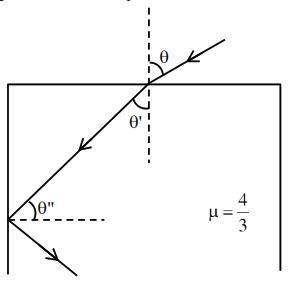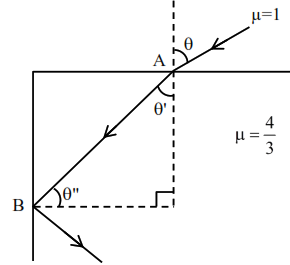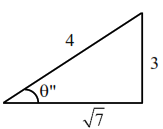# A ray of light enteringQuestion:

A ray of light entering from air into a denser medium of refractive index $\frac{4}{3}$, as shown in figure. The light ray suffers total internal reflection at the adjacent surface as shown. The maximum value of angle $\theta$ should be equal to :1. $\sin ^{-1} \frac{\sqrt{7}}{3}$

2. $\sin ^{-1} \frac{\sqrt{5}}{4}$

3. $\sin ^{-1} \frac{\sqrt{7}}{4}$

4. $\sin ^{-1} \frac{\sqrt{5}}{3}$

Correct Option: 1

Solution:At maximum angle $\theta$ ray at point B goes in gazing emergence, at all less values of $\theta$, TIR occurs.

At point B

$\frac{4}{3} \times \sin \theta^{\prime \prime}=1 \times \sin 90^{\circ}$

$\theta^{\prime \prime}=\sin ^{-1}\left(\frac{3}{4}\right)$

$\theta^{\prime}=\left(\frac{\pi}{2}-\theta^{\prime \prime}\right)$

At point $\mathrm{A}$

$1 \times \sin \theta=\frac{4}{3} \times \sin \theta^{\prime}$

$\sin \theta=\frac{4}{3} \times \sin \left(\frac{\pi}{2}-\theta^{\prime \prime}\right)$

$\sin \theta=\frac{4}{3} \cos \left[\cos ^{-1} \frac{\sqrt{7}}{4}\right]$$\sin \theta=\frac{4}{3} \times \frac{\sqrt{7}}{4}$

$\theta=\sin ^{-1}\left(\frac{\sqrt{7}}{3}\right)$